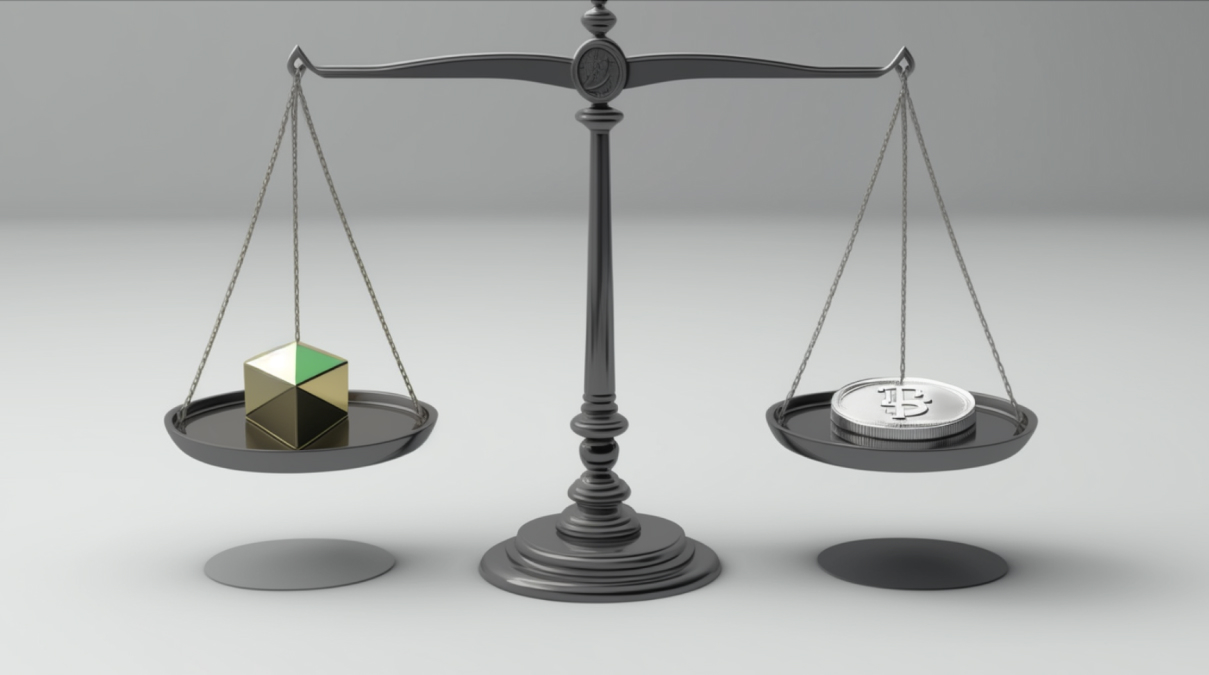STC Educational Panel

## Volume-Weighted Average Price (VWAP)

Intermediate
March 24, 2023
6mVolume-Weighted Average Price (VWAP) is the average price of an asset, taking into account both volume and price. It is used to determine the overall direction of the cryptocurrency market and as a market analysis indicator to provide insight into trends and values.

This indicator is often used by individual traders and financial institutions that deal with large quantities of assets.

#### How is the Volume-Weighted Average Price calculated?

VWAP is usually used for one-day or shorter periods, depending on needs.

The indicator is calculated by totaling the dollars traded for every transaction in a period (price x volume) and dividing the total trading volume for the given period.

Here are 5 steps to calculate VWAP:

Determine the period over which the VWAP will be calculated.

Determine the trading volume for the asset over the selected period. This can be obtained from exchange data or trading charts.

Calculate the cumulative price-volume product for each period within the time frame. Multiply the asset price by the trading volume for that period, then add up all of these values to get the cumulative amount.

Calculate the cumulative trading volume, by adding up the trading volume for each period within the time frame to get the cumulative trading volume.

Finally,
calculate VWAP.

The formula for calculating VWAP is as follows:
VWAP = Cumulative Typical Price x Volume/Cumulative Volume

where:
Cumulative – total in a given period
Typical Price – according to the formula – Typical Price = High price + Low price + Closing Price/3
Volume – the amount of units of an asset sold or bought in a given transaction

The result of the above calculations represents the transaction's price, considering the volume (the weighted average price of transactions made over a certain period).

#### How important is the VWAP for traders?

VWAP, if used correctly, can become an essential tool for traders. By determining the volume-weighted average price, they can compare the result obtained with the asset's current market price and, on this basis, assess whether the current price represents the right opportunity to buy.

#### Example of use

Let's assume we decide to purchase large amounts of cryptocurrency X, which is gaining popularity in the market. However, this is a new asset for us, and we are still determining what price might be a good buying opportunity. We can check the asset's current market price and compare it with the VWAP value.

If the price is above the VWAP value, the market is bullish.

Whereas when the price is below the VWAP value, the market is bearish, which may signal a favorable price and the right time to execute a purchase.

#### Summary

VWAP is the volume-weighted average price of trades that took place in a given time frame. It is used by traders to analyze the current market price and compare it to the value of the weighted average. VWAP makes it possible to assess whether a given market price is favorable enough to invest in.

Complete quiz
Volume-Weighted Average Price (VWAP)Beginner

### What is an NFT (Non-Fungible Token)?

NFTs redefine asset ownership and allow the tokenization of both digital and physical items. Since they are created on a blockchain and stored in digital wallets, they can be easily traded, sold, and bought transparently and efficiently.Beginner

### What is Market Liquidity?

Market liquidity refers to the ease and speed of buying and selling a particular cryptocurrency and is determined by the demand and supply of the asset.Intermediate

### Halving

Halving is a process in which the reward for mining a cryptocurrency is cut in half. At the same time, it reduces the asset’s supply in the market. Halving can affect the price, mining process, and security.